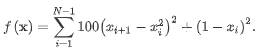# How To Make Superfast Scientific Calculations With SciPy And DelphiDo you want to build a GUI App with Scientific Computation capabilities? In this post will get to understand how to use SciPy Python Library using Python4Delphi in Delphi/C++ application. SciPy is a collection of mathematical algorithms and convenience functions built on the NumPy extension of Python.

SciPy is organized into sub packages covering different scientific computing domains such as cluster, special, integrate, optimize, interpolate, linalg etc.

Python for Delphi (P4D) is a set of free components that wrap up the Python DLL into Delphi and Lazarus (FPC). They let you easily execute Python scripts, create new Python modules and new Python types. You can use Python4Delphi a number of different ways such as:

• Create a Windows GUI around your existing Python app.
• Enhance your speed-sensitive Python apps with functions from Delphi for more speed.

## What are the prerequisites for using SciPy and Delphi?

• If not python and Python4Delphi is not installed on your machine, Check this how to run a simple python script in Delphi application using Python4Delphi sample app
• Open windows open command prompt, and type pip install -U SciPy to install SciPy. For more info for Installing Python Modules check here
• First, run the Demo1 project for executing Python script in Python for Delphi. Then load the SciPy sample script in the Memo1 field and press the Execute Script button to see the result. On Clicking Execute Button the script strings are executed using the below code. Go to GitHub to download the Demo1 source.

## What does the example of using the Scipy Python Library look like?

Here are the Scipy Python Library sample script details. It covers some of the SciPy sub packages functions such as Bessel, Integration, Interpolation, and optimization.

## What does the SciPy.Special subpackage do?

• The main feature of the `scipy.special` package is the definition of numerous special functions of mathematical physics. Available functions include airy, elliptic, bessel, gamma, beta, hypergeometric, parabolic cylinder, mathieu, spheroidal wave, struve, and kelvin.
• Bessel functions are a family of solutions to Bessel’s differential equation with real or complex order alpha. These functions arise in wave propagation problems, such as the vibrational modes of a thin drum head. Here is an example of a circular drum head anchored at the edge:

## What is the SciPy.Integrate Subpackage?

• The `scipy.integrate` sub-package provides several integration techniques including an ordinary differential equation integrator.
• The function quad is provided to integrate a function of one variable between two points. For example, to integrate a bessel function `jv(2.5, x)` along the interval [0,4.5].This could be computed using `quad` and the result as shown in Memo output.

## What is the SciPy.Optimize subpackage and what does it do?

• The `scipy.optimize` package provides several commonly used optimization algorithms. To demonstrate the minimization function, consider the problem of minimizing the Rosenbrock function of N variables:The minimum value of this function is 0 which is achieved when xi=1.
• The `minimize` routine is used with the Nelder-Mead simplex algorithm (selected through the `method` parameter) and the result as shown in Memo output.

## What is the function of the SciPy.Interpolate subpackage?

• The `interp1d` class in `scipy.interpolate` is a convenient method to create a function based on fixed data points, which can be evaluated anywhere within the domain defined by the given data using linear interpolation. This example demonstrates its use, for linear and cubic spline interpolation:

Note: Samples used for demonstration were picked from here with only the difference of printing the outputs. You can check the APIs and some more samples from the same place.

You have read the quick overview of SciPy library, download this library from here and use user-friendly scientific computation functions in your applications. Check out Python4Delphi and easily build Python GUIs for Windows using Delphi.